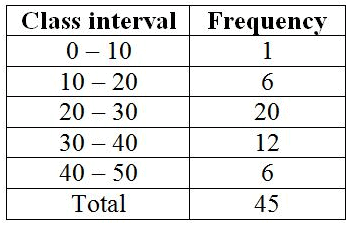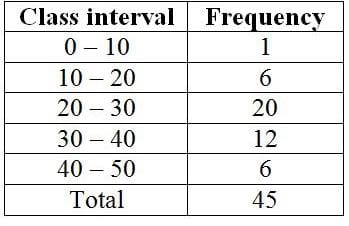Test: Data Handling

# Test: Data Handling

Test Description

## 10 Questions MCQ Test Mathematics (Maths) Class 7 | Test: Data Handling

Test: Data Handling for Class 7 2022 is part of Mathematics (Maths) Class 7 preparation. The Test: Data Handling questions and answers have been prepared according to the Class 7 exam syllabus.The Test: Data Handling MCQs are made for Class 7 2022 Exam. Find important definitions, questions, notes, meanings, examples, exercises, MCQs and online tests for Test: Data Handling below.
Solutions of Test: Data Handling questions in English are available as part of our Mathematics (Maths) Class 7 for Class 7 & Test: Data Handling solutions in Hindi for Mathematics (Maths) Class 7 course. Download more important topics, notes, lectures and mock test series for Class 7 Exam by signing up for free. Attempt Test: Data Handling | 10 questions in 10 minutes | Mock test for Class 7 preparation | Free important questions MCQ to study Mathematics (Maths) Class 7 for Class 7 Exam | Download free PDF with solutions
 1 Crore+ students have signed up on EduRev. Have you?
Test: Data Handling - Question 1

### The number of times an observation occurs in a data is called its

Detailed Solution for Test: Data Handling - Question 1

Data available in an unorganised form are called raw data. The number of times a particular observation occurs in a given data is called its frequency.

Test: Data Handling - Question 2

### In the class- interval 70-80, 80 is the

Detailed Solution for Test: Data Handling - Question 2

Upper limit is the largest quantity in the interval. So 80 is the upper limit

Test: Data Handling - Question 3

### The class mark of class 95- 100 is

Detailed Solution for Test: Data Handling - Question 3

Class mark is the midpoint of the upper and lower limits.
so for the given problem; the class mark is
[(95+100)÷2] = 97.5
Thus class mark is 97.5

So option C is the correct answer.

Test: Data Handling - Question 4

The difference between the upper and lower limit is called

Detailed Solution for Test: Data Handling - Question 4

The difference between the upper and lower limit of a class interval is called the class size of the class interval.

Test: Data Handling - Question 5

The mean weight of 100 students in a class is 46 kg. The mean weight of boys is 50 and of girls is 40 kg. Therefore, the number of boys is:

Detailed Solution for Test: Data Handling - Question 5

Let number of boys are x and then number of girls = (100-x).
Thus,
50x+(100-x)*40 = 46*100
→ x = 60.
Number of boys = 60.

Test: Data Handling - Question 6

Find the mean weight of the data set shown. 5 kg, 43 kg, 18 kg, 26 kg, 28 kg, 46 kg, 41 kg, 1 kg

Detailed Solution for Test: Data Handling - Question 6

Mean =  Sum of mass weights / Total number of weighs

= (5 + 43 + 18 + 26 + 28 + 46 + 41 + 1)/8

= 26

Test: Data Handling - Question 7

A kennel can accommodate 12 dogs that weigh (in pounds) 6, 14, 23, 17, 19, 27, 39, 7, 33, 4, 11, and 13. Find the range of their weights.

Detailed Solution for Test: Data Handling - Question 7

The Range is the difference between the lowest and highest values.

Here the highest value is 39 and the lowest value is 4

So Range = Highest Value - Lowest Value

= 39 - 4 = 35

So option B is the correct answer.

Test: Data Handling - Question 8

Find the mode for the data set, which shows the heights (in inches) of 10 students of Piyush’s class. 65, 60, 64, 61, 66, 67, 67, 67, 62, 77

Detailed Solution for Test: Data Handling - Question 8

the mode of a set of numbers is the number that appears most often in the set.

Since the number 67 appears the most . So the mode of the given data is 67.

So option B is the correct answer.

Test: Data Handling - Question 9

Following frequency distribution table shows marks (out of 50) obtained in English by 45 students of class VII. What is the size of class intervals?Test: Data Handling - Question 10

Which class has the highest frequency?## Mathematics (Maths) Class 7

168 videos|252 docs|45 tests
 Use Code STAYHOME200 and get INR 200 additional OFF Use Coupon Code
Information about Test: Data Handling Page
In this test you can find the Exam questions for Test: Data Handling solved & explained in the simplest way possible. Besides giving Questions and answers for Test: Data Handling, EduRev gives you an ample number of Online tests for practice

## Mathematics (Maths) Class 7

168 videos|252 docs|45 tests